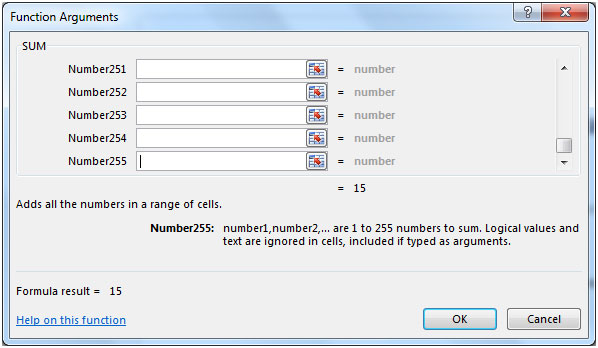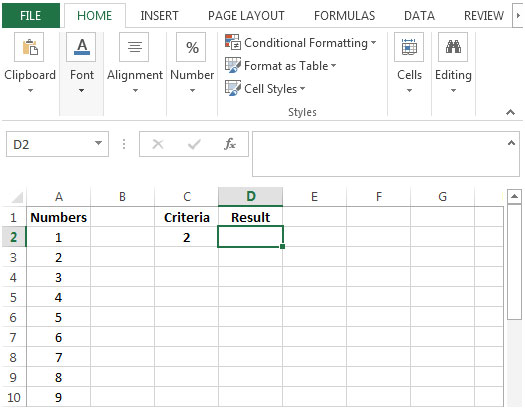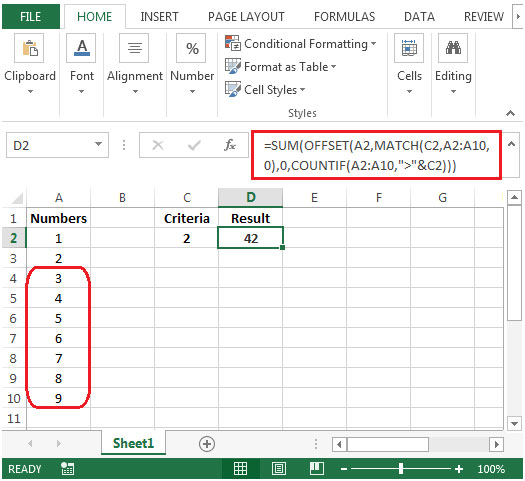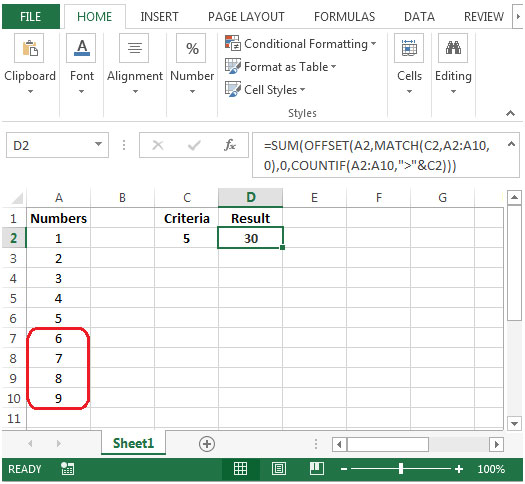# Summing A Dynamic Range of Numbers According To Criteria in Excel 2010

To find sum of numbers based on criteria, we can use a combination of SUM, MATCH, COUNTIF & OFFSET functions to retrieve the output.

SUM: Adds all the numbers in a range of cells

Syntax: =SUM(number1,number2,...)

There can be maximum 255 arguments. Refer below shown screenshot:MATCH function searches for a specified item in a selected range of cells, and then returns the relative position of that item in the range.

Syntax =MATCH(lookup_value,lookup_array,match_type)

COUNTIF: Counts the number of cells within a range that meets the condition.

Syntax: =COUNTIF(range,criteria)

OFFSET: Returns a reference to a range that is a given number of rows and columns from a given reference.

Syntax: =OFFSET(reference,rows,cols,height,width)

reference: It is a reference to a cell or range of cells from which the offset will be applied.

rows: The number of rows up or down that you want to apply as the offset to the range.

cols: The number of columns left or right that you want to apply as the offset to the range.

height: This is optional. It is the number of rows that you want the returned reference to be.

width: This is optional. It is the number of columns that you want the returned reference to be.

Let us take an example:

Column A contains an ascending list of numbers. We need a formula that will sum up all the numbers in column A larger than 2 which is our criterion in cell C2.• The formula in cell D2 would be
• =SUM(OFFSET(A2,MATCH(C2,A2:A10,0),0,COUNTIF(A2:A10,">"&C2)))• The formula has taken the sum of numbers which are greater than 2.
• If we change the criteria in cell C2 from 2 to 5 then the result would be updated automatically. This time formula will calculate sum of the numbers which are greater than 5.1.How to get the sum of values with multiple values in criteria column.
For Eg: I want the sum of all the values which are equal to the list in column C "Criteria"
And Criteria will have 2, 5, 6 and 7

•You can do this using curly braces when defining criteria. `=SUMIF(C:C,{2,5,6,7}, sum_range)`. Use CTRL+SHIFT+Enter once you have written the formula.
Or you can use the sumproduct function to do so. Here I have explained it. https://www.exceltip.com/summing/sum-if-with-sumproduct-and-or-criteria-in-excel.html

Terms and Conditions of use

The applications/code on this site are distributed as is and without warranties or liability. In no event shall the owner of the copyrights, or the authors of the applications/code be liable for any loss of profit, any problems or any damage resulting from the use or evaluation of the applications/code.